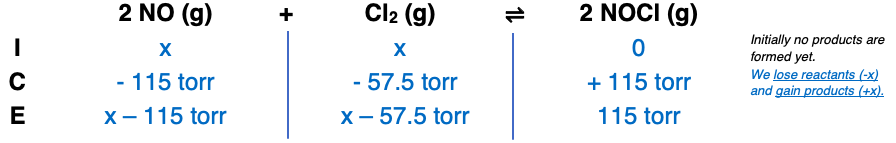# Problem: Nitric oxide reacts with chlorine gas according to the following reaction: 2NO(g) + Cl2(g) ⇌ 2NOCl(g); Kp = 0.27 at 700 KA reaction mixture initially contains equal partial pressures of  NO and Cl2. At equilibrium, the partial pressure of NOCl was measured to be 115 torr. What were the initial partial pressures of NO and Cl2?

###### FREE Expert Solution88% (111 ratings)###### Problem Details

Nitric oxide reacts with chlorine gas according to the following reaction: 2NO(g) + Cl2(g) ⇌ 2NOCl(g); Kp = 0.27 at 700 K
A reaction mixture initially contains equal partial pressures of  NO and Cl2. At equilibrium, the partial pressure of NOCl was measured to be 115 torr. What were the initial partial pressures of NO and Cl2?